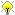[Documentation] [TitleIndex] [WordIndex]

 Note: This tutorial assumes you have completed the tf and time (Python) (C++) tutorials.Please ask about problems and questions regarding this tutorial on answers.ros.org. Don't forget to include in your question the link to this page, the versions of your OS & ROS, and also add appropriate tags.

# Time travel with tf (C++)

Description: This tutorial teaches you about advanced time travel features of tf

In the previous tutorial we discussed the basic concept of tf and time. This tutorial will take this one step further, and expose one of the most powerful tf tricks.

## Time travel

So let's go back to where we ended in the previous tutorial. Go to your package for the tutorial:

`  \$ roscd learning_tf`

and open the file src/turtle_tf_listener.cpp. Take a look at lines 25-30:

```  try{
ros::Time now = ros::Time::now();
listener.waitForTransform("/turtle2", "/turtle1",
now, ros::Duration(1.0));
listener.lookupTransform("/turtle2", "/turtle1",
now, transform);```

Now, instead of making the second turtle go to where the first turtle is now, make the second turtle go to where the first turtle was 5 seconds ago:

```  try{
ros::Time past = ros::Time::now() - ros::Duration(5.0);
listener.waitForTransform("/turtle2", "/turtle1",
past, ros::Duration(1.0));
listener.lookupTransform("/turtle2", "/turtle1",
past, transform);```

So now, if you would run this, what would you expect to see? Definitely during the first 5 seconds the second turtle would not know where to go, because we do not yet have a 5 second history of the first turtle. But what after these 5 seconds? Let's just give it a try:

```  \$ make  or  catkin_make
\$ roslaunch learning_tf start_demo.launch```
•Is your turtle driving around uncontrollably like in this screenshot? So what is happening?

• We asked tf, "What was the pose of /turtle1 5 seconds ago, relative to /turtle2 5 seconds ago?". This means we are controlling the second turtle based on where it was 5 seconds ago as well as where the first turtle was 5 seconds ago.

• What we really want to ask is, "What was the pose of /turtle1 5 seconds ago, relative to the current position of the /turtle2?".

So how can we ask tf a question like that? This API gives us the power to say explicitly when each frame is transformed. This is what the code would look like:

```  try{
ros::Time now = ros::Time::now();
ros::Time past = now - ros::Duration(5.0);
listener.waitForTransform("/turtle2", now,
"/turtle1", past,
"/world", ros::Duration(1.0));
listener.lookupTransform("/turtle2", now,
"/turtle1", past,
"/world", transform);```

The advanced API for lookupTransform() takes six arguments:

1. Give the transform from this frame,
2. at this time ...
3. ... to this frame,
4. at this time.
5. Specify the frame that does not change over time, in this case the "/world" frame, and
6. the variable to store the result in.

Notice that waitForTransform() also has a basic and and advanced API, just like lookupTransform().

•This figure shows what tf is doing in the background. In the past it computes the transform from the first turtle to the world. In the world frame tf time travels from the past to now. And at time now tf computes the transform from the world to the second turtle.

## Checking the results

Let's run the simulator again, this time with the advanced time-travel API:

```  \$ make  or catkin_make
\$ roslaunch learning_tf start_demo.launch```

And yes, the second turtle is directed to where the first turtle was 5 seconds ago!

2020-01-18 13:14